# Weka: A Free and Open Source Suite for Machine Learning and Deep Learning Algorithms

0
5488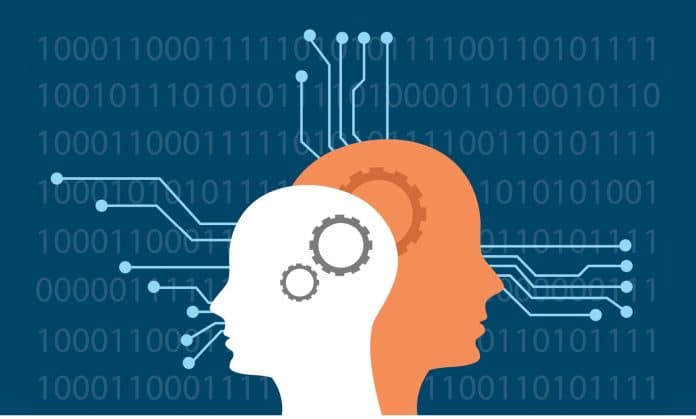Weka or the Waikato Environment for Knowledge Analysis is a machine learning suite that is written in Java, having been developed by the University of Waikato in New Zealand. This article is an introduction to an effective ML tool.

Machine learning, deep learning and predictive analytics are the key domains of research in engineering, finance, economics, real-time imaging and many other fields. Researchers are working on different tools and technologies in these fields so that a higher degree of accuracy can be achieved.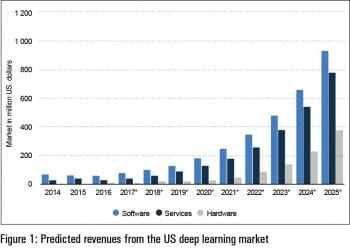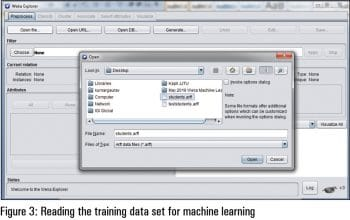The deep learning industry is very closely integrated with machine learning (ML), resulting in a higher degree of performance and accuracy with the minimum error rate. Figure 1 gives the predicted CAGR in revenues from deep learning in the USA, during the period 2014 to 2025.

Weka is a free and open source tool for machine learning and Big Data analytics (URL: https://www.cs.waikato.ac.nz/ml/weka/). Table 1 lists the prominent tools and software libraries used for the machine learning and data science based implementations.

Although there are a number of software libraries being widely used, Weka is a powerful tool preferred by researchers and data scientists. It has a huge set of machine learning and data science based algorithms including Big Data analytics. Weka can be used with the command line interface as well as the graphical user interface (GUI) for the implementation of algorithms. Besides the inbuilt and pre-loaded packages in Weka, there are assorted extension packages that can be integrated for advanced applications.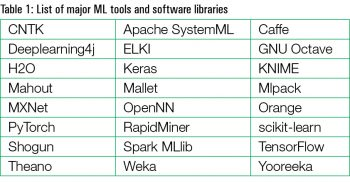Predictions using machine learning algorithms in Weka
Let us look at an example of a classifier based machine learning algorithm being used in Weka. For this, the classification problem of the data set of students is used. In this example, the data set Students.arffis is used for training the classifier model. The marks of students are given in three phases. On the basis of marks obtained in sequence, the final class attribute is determined. In the first record, if a student scored 90, 89 and 89 marks, respectively, then the student was given admission to Stream 1. In the third record, Stream 2 is allocated to the students who obtained marks 78, 67 and 78, respectively.

```Students.arff
@relation students
@attribute marks1 numeric
@attribute marks2 numeric
@attribute marks3 numeric
@attribute class {1, 2}
@data
90, 89, 89, 1
89, 90, 99, 1
78, 67, 78, 2
67, 71, 78, 2
69, 78, 78, 2
60, 79, 78, 2```

The test data set teststudents.arff is used to predict or determine the class or stream of the students who obtained marks in a specific sequence. This problem is solved using Weka with the integration of the machine learning algorithm of J48 classifier. In the following data set, we have to determine the classes (streams) of the students on the basis of their performance (scores) in the examination.

```teststudents.arff
@relation students
@attribute marks1 numeric
@attribute marks2 numeric
@attribute marks3 numeric
@attribute class {1, 2}
@data
99, 91, 90, ?
89, 67, 78, ?
78, 67, 78, ?
77, 71, 78, ?
90, 78, 78, ?
10, 10, 10, ?
40, 40, 78, ?
30, 30, 80, ?
98, 97, 94, ?```

Figure 2 depicts the Weka GUI Chooser interface in which there are multiple options to work with the data science and machine learning based implementations. In this option, there are multiple options including Explorer, Experimenter, Knowledge Flow, Workbench and Simple CI. For traditional implementations, Explorer is used by data scientists, as it has user friendly interfaces to choose the data set and apply different algorithms without cramming any instructions or syntax for the algorithmic implementation.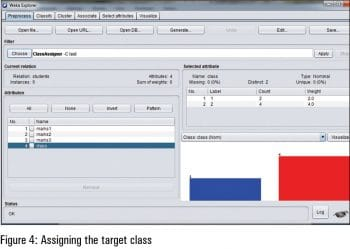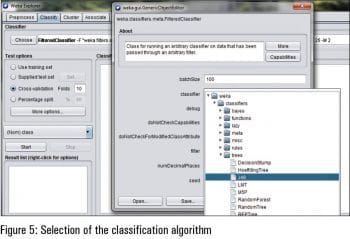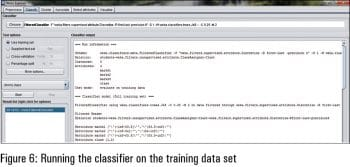From the Preprocess Tab in Weka, as shown in Figure 3, the training data can be selected. In the option to open the file, the data scientist can select the data set that is required to be trained for the modelling and processing of the classifier, as per the current scenario.

Once the training data set is selected and imported to the Weka interface, the target class is required to be mentioned. As per the training data, the attribute ‘class’ is used here as the target. It means that the ‘class’ is the determined value on the combinations and associations of other attributes — marks1, marks2 and marks3.

In Weka, there are assorted algorithms for data science and machine learning that can be called and attached with the data set to be processed. Figure 5 presents the option to select the classification algorithm of J48 so that the classification model can be built on the basis of the training data selected earlier.

Once the data set and classification model are invoked, there is a need to run the classifier so that the model can be trained. In this way, the classification model is correlated with the association of determining attributes and the determined attribute. In this example of students, the determining attributes are marks1, marks2 and marks3. The determined attribute or target is the class that has an association with the determining attributes or dependent attributes in the training data set.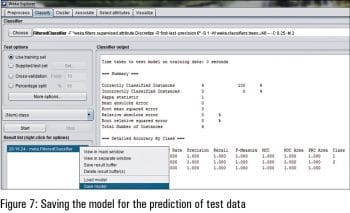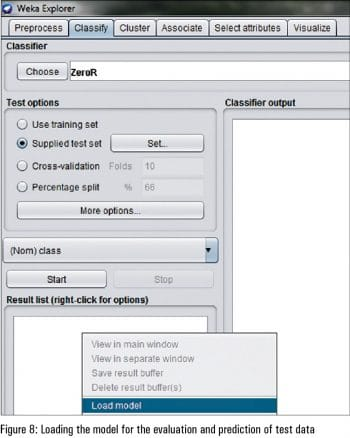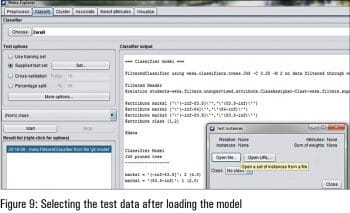The classification algorithm for machine learning is executed and then has to be saved so that the prediction on the testing or validation data set can be done. This means that the trained model has the association functions and the mathematical modelling of all the attributes. These mathematical modelling functions are further required for the prediction of test data that does not have the classes. The predicted class of the testing or validation data is determined on the functions created by the trained classification model as per the algorithm executed.

For the prediction of the training data set, the test data set is matched with the saved model. The saved classification model is loaded in the Weka panel and then the option of ‘Supplied test set’ is used for testing data. The testing data set is called using the ‘Set’ option so that it can be predicted with the saved classification model.

After loading the saved classification model of machine learning, the test (validation) data set is read so that the unknown classes (represented as ‘?’ ) in the testing data can be predicted.

To view the predicted classes, the option of Output Predictions’ in the ‘Classifier Evaluation Options’ is set to ‘PlainText’ so that the unknown classes can be viewed on the Weka interface.
After loading the saved model and invoking the testing data set, the re-evaluation of the model is done specifically for the supplied test set. It is used so that the supplied testing data set can be assigned the predicted classes on the same mathematical functions and model as used in the earlier classification model.

The predicted classes can be viewed in the right side panel of Weka after re-evaluation of the model. As in Figure 12, there are multiple attributes including the instance number, actual and predicted. In the predicted attribute, the unknown class (represented as‘?’) is assigned with the determined class on the same algorithm of machine learning. For example, in the first instance, the predicted class is 1, which was unknown in the test data set. In a similar way, the other values can be predicted.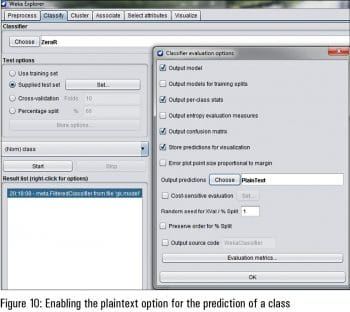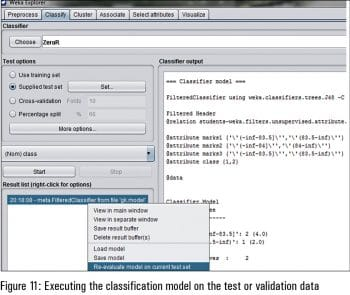Using WekaDeeplearning4j for deep learning in Weka
WekaDeepLearning4j (https://deeplearning.cms.waikato.ac.nz/) is the dedicated package for the implementation of deep learning in different applications. This library has been released so that the features and accuracy of deep learning can be used with the data analytics and predictive mining based applications. The power of Java programming is associated at the back-end of Weka with deep learning modules.
The following are the key features and layers integrated in Weka with deep learning:

• Convolution layer
• Dense layer
• Sub-sampling layer
• Batch normalisation
• Long short term memory (LSTM)
• Global pooling layer
• Output layer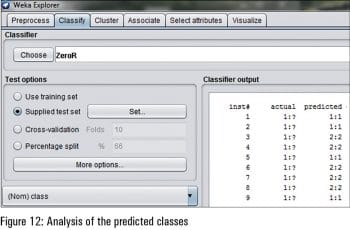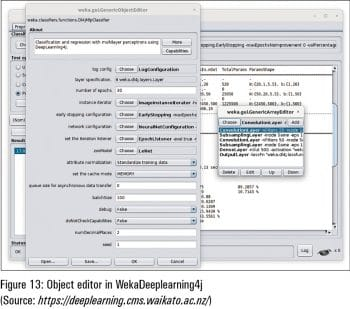Scope for research and development
The algorithms of machine learning, deep learning, data science and knowledge discovery are closely associated with scientific and engineering applications. They can be used for the development of new algorithms and for solving the engineering optimisation problems in the social as well as scientific domains. These algorithms have custom functions that can be updated as per the requirements of dynamic data sets to achieve a higher degree of accuracy and performance with related degrees of effectiveness.The author is the managing director of Magma Research and Consultancy Pvt Ltd, Ambala Cantonment, Haryana. He has 16 years experience in teaching, in industry and in research. He is a projects contributor for the Web-based source code repository SourceForge.net. He is associated with various central, state and deemed universities in India as a research guide and consultant. He is also an author and consultant reviewer/member of advisory panels for various journals, magazines and periodicals. The author can be reached at kumargaurav.in@gmail.com.